# How to Calculate and Solve for Specific Storage | Aquifer Characteristics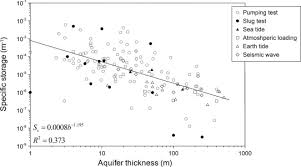The image above represents specific storage.

To compute for specific storage, five essential parameters are needed and these parameters are Density of Water (ρw), Acceleration due to gravity (g), Compressibility of the Aquifer (α), Porosity (n) and Compressibility of Water (β).

The formula for calculating specific storage:

Ss = ρwg(α + nβ)

Where:

Ss = Specific Storage
ρw = Density of Water
g = Acceleration due to Gravity
α = Compressibility of the Aquifer
n = Porosity
β = Compressibility of Water

Let’s solve an example;
Find the specific storage when the density of water is 22, the acceleration due to gravity is 9.8, the comepressibility of the aquifer is 15, the porosity is 10 and the compressibility of water is 22.

This implies that;

ρw = Density of Water = 22
g = Acceleration due to Gravity = 9.8
α = Compressibility of the Aquifer = 15
n = Porosity = 10
β = Compressibility of Water = 22

Ss = ρwg(α + nβ)
Ss = (22)(9.8)(15 + 10(22))
Ss = 215.60 (15 + 220)
Ss = 215.60 (235)
Ss = 50666.0

Therefore, the specific storage is 50666.0.

Nickzom Calculator – The Calculator Encyclopedia is capable of calculating the specific storage.

To get the answer and workings of the specific storage using the Nickzom Calculator – The Calculator Encyclopedia. First, you need to obtain the app.

You can get this app via any of these means:

To get access to the professional version via web, you need to register and subscribe for NGN 2,000 per annum to have utter access to all functionalities.
You can also try the demo version via https://www.nickzom.org/calculator

Apple (Paid) – https://itunes.apple.com/us/app/nickzom-calculator/id1331162702?mt=8
Once, you have obtained the calculator encyclopedia app, proceed to the Calculator Map, then click on Geology under Add-on.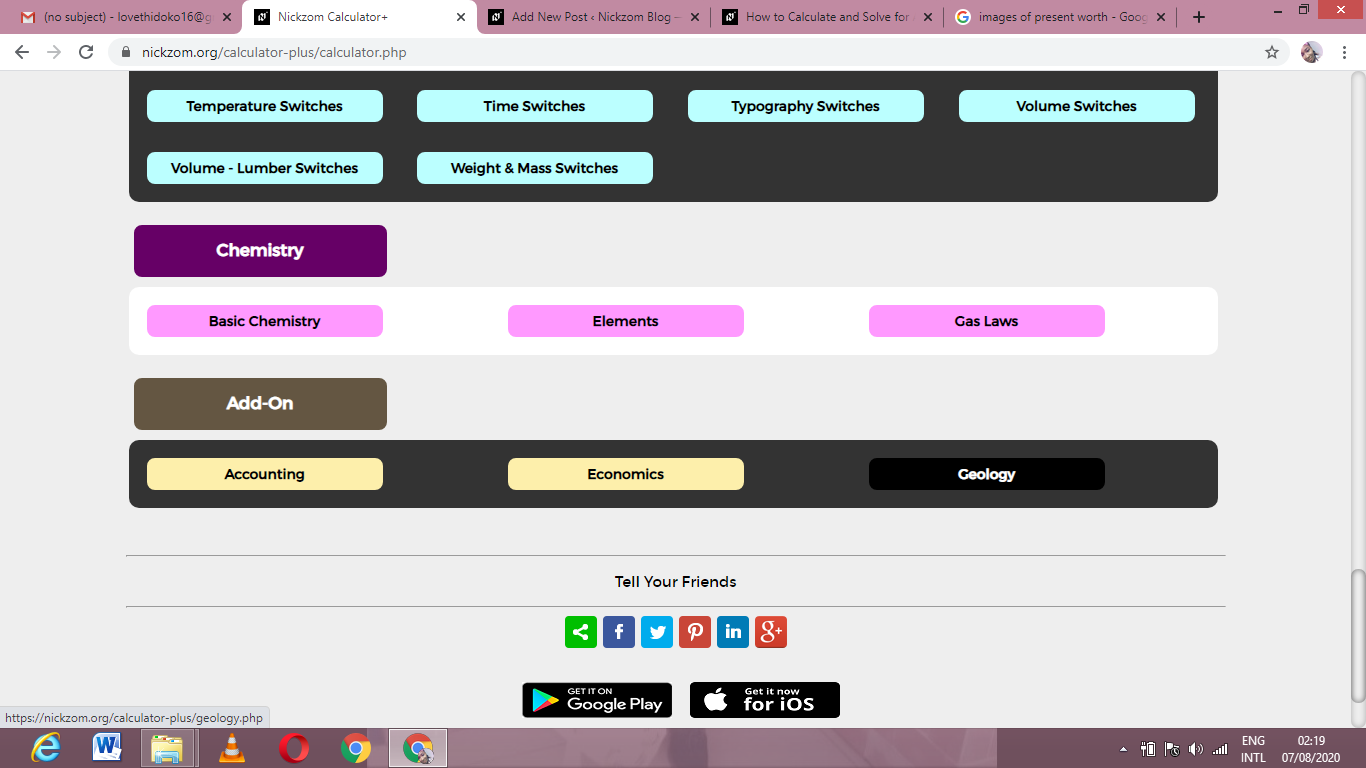Now, Click on Aquifer Characteristics under GeologyNow, Click on Specific Storage under Aquifer Characteristics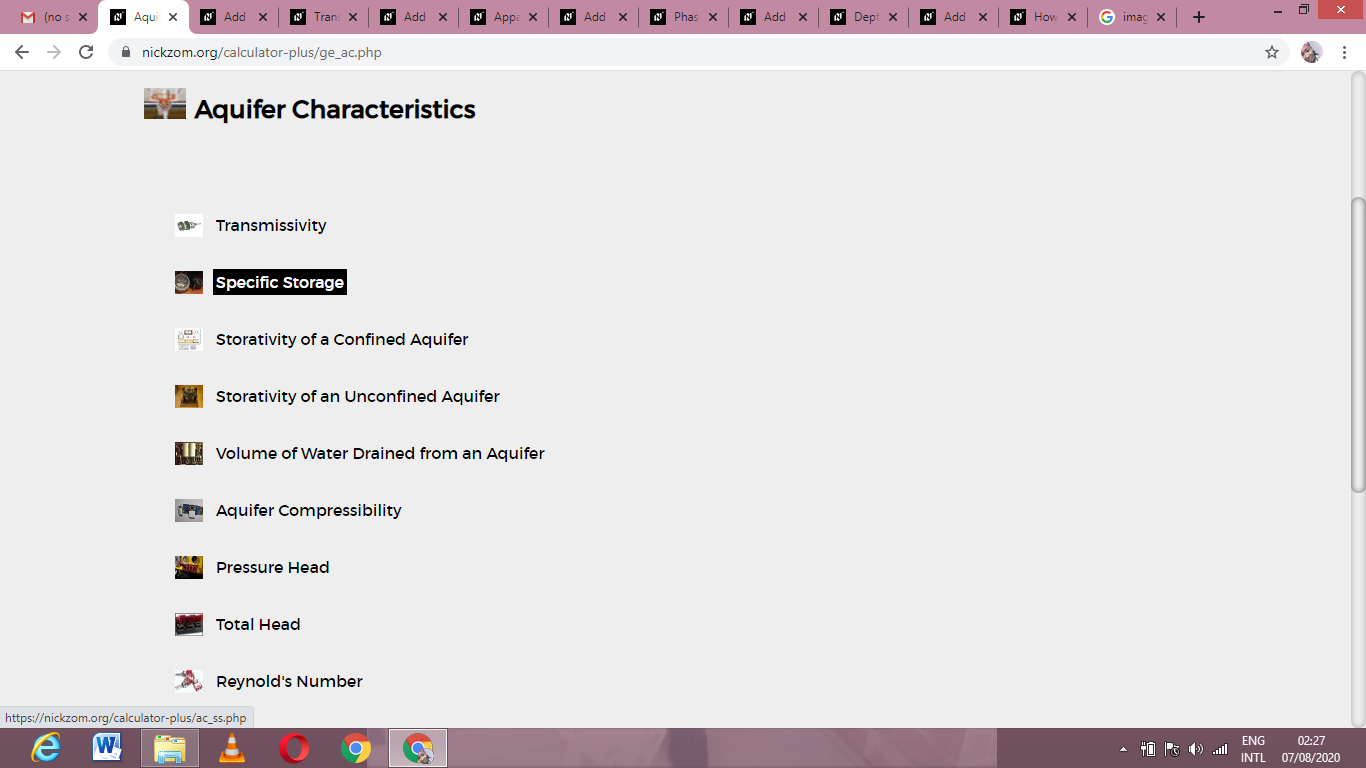The screenshot below displays the page or activity to enter your values, to get the answer for the specific storage according to the respective parameters which are the Density of Water (ρw), Acceleration due to gravity (g), Compressibility of the Aquifer (α), Porosity (n) and Compressibility of Water (β).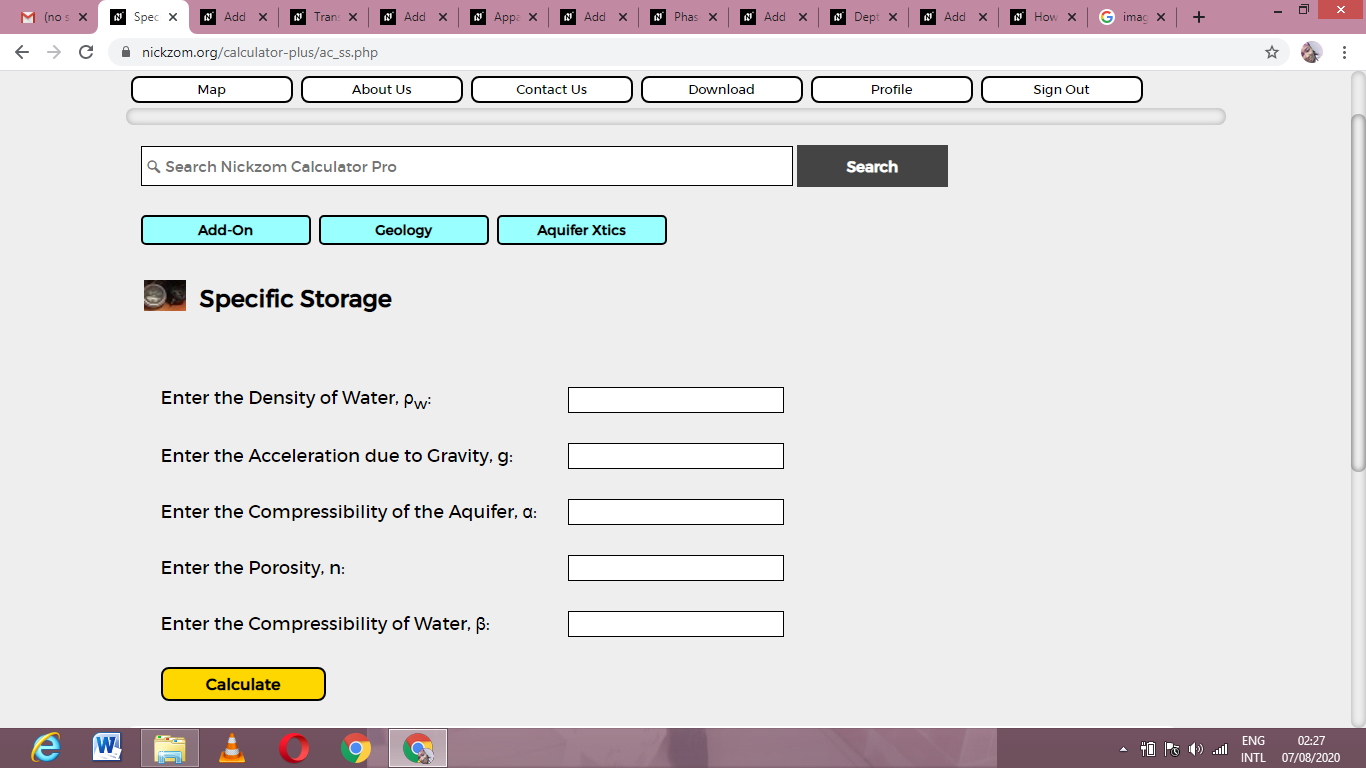Now, enter the values appropriately and accordingly for the parameters as required by the Density of Water (ρw) is 22, Acceleration due to gravity (g) is 9.8, Compressibility of the Aquifer (α) is 15, Porosity (n) is 10 and Compressibility of Water (β) is 22.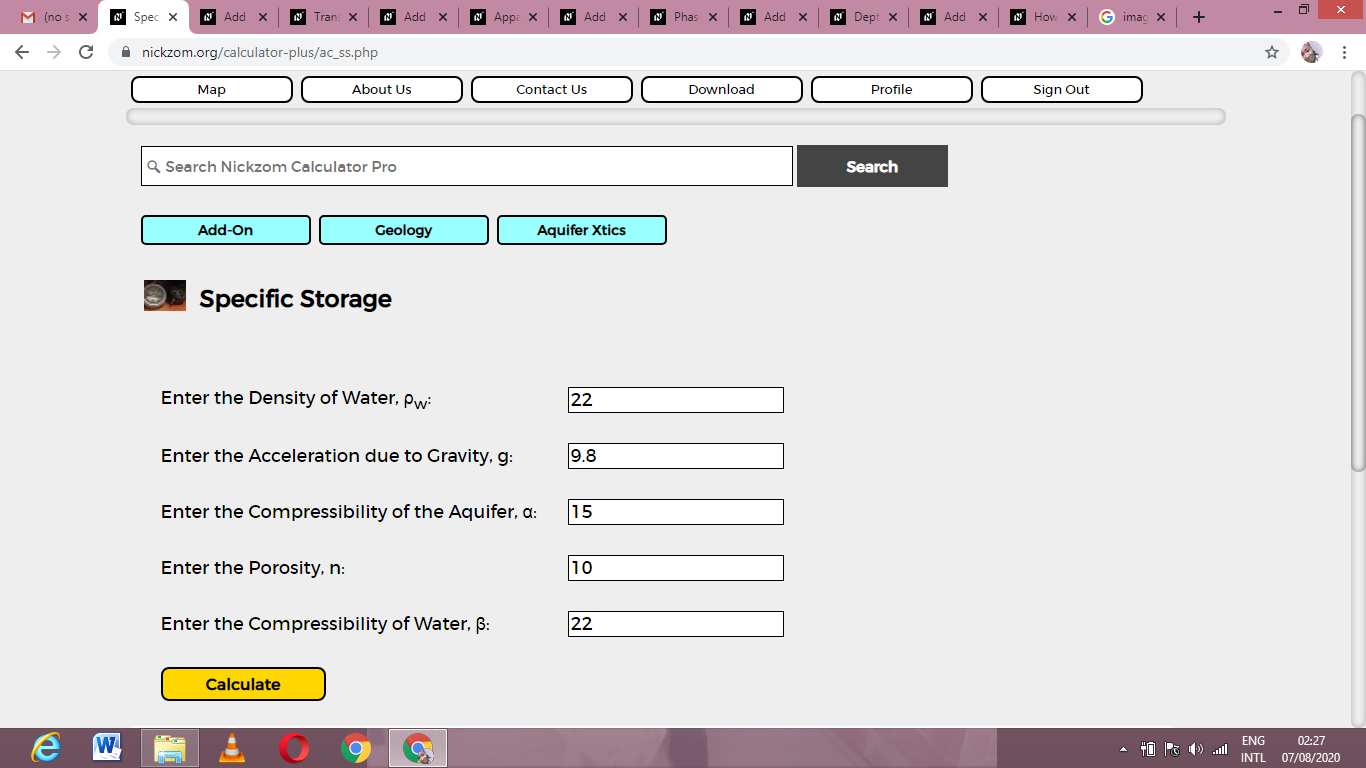Finally, Click on Calculate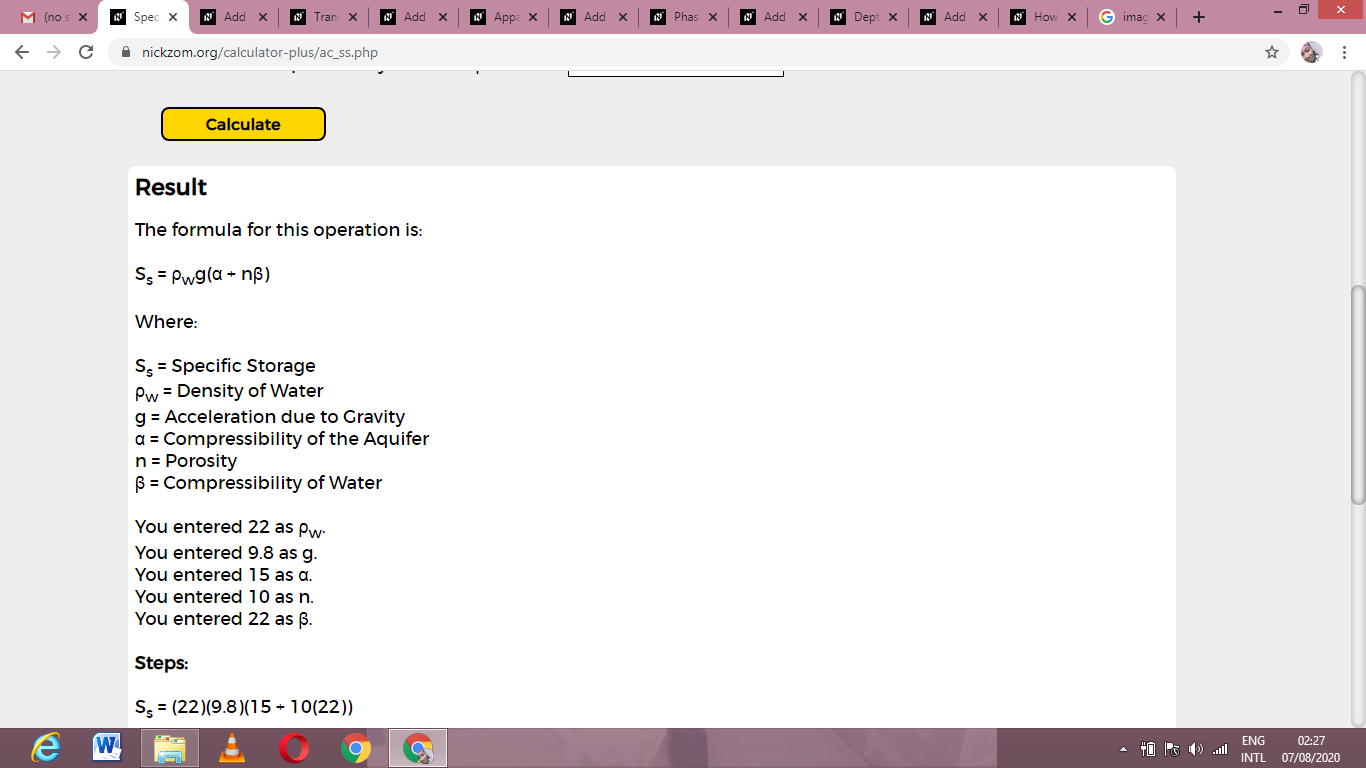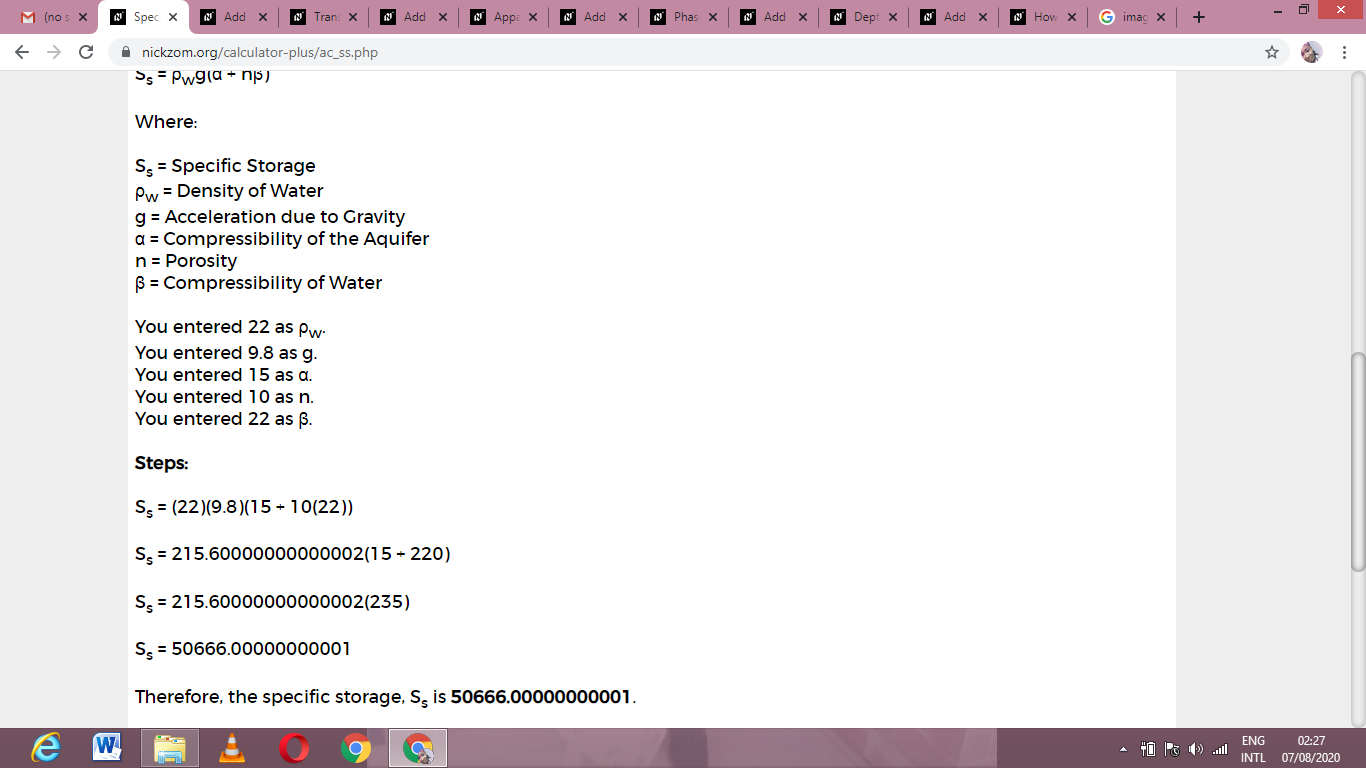As you can see from the screenshot above, Nickzom Calculator– The Calculator Encyclopedia solves for the specific storage and presents the formula, workings and steps too.